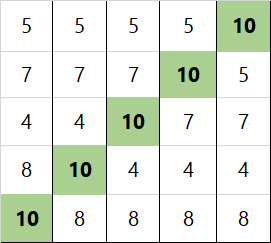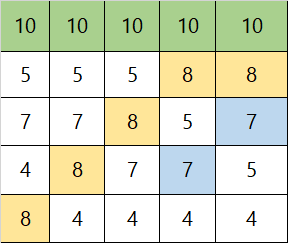# Python 实现冒泡排序

li = [10,8,4,7,5]


#### 内层循环：for j in range(len(li)-1):      # 列表中一个5个数，比较为两两比较，因此实际次数为4次
if li[j] > li[j + 1]:       # 判断前面的数是否大于后面的数
li[j], li[j + 1] = li[j + 1], li[j]   #大于则交换位置


len(li) - 1：len(li) 获取列表li中的元素个数；这里我们只进行一次循环的情况，循环的次数为元素的个数 -1；
li[j] > li[j + 1]：就是比较大小啦；
li[j], li[j + 1] = li[j + 1], li[j]：Python 中的多元赋值，也是一种很方便的交换变量值的方式，省去了中间变量。

li[j], li[j + 1] = li[j + 1], li[j]


temp = li[j]
li[j] = li[j+1]
li[j+1]=tempfor j in range(len(li)-1-i):      # i为一个递增的数，减去递增，就是递减嘛


#### 外层循环：

for i in range(len(li)-1):      # n-1次冒泡


#### 最终结果：

li = [10,8,4,7,5]
for i in range(len(li)-1):
for j in range(len(li)-1-i):
if li[j] > li[j + 1]:
li[j], li[j + 1] = li[j + 1], li[j]
print(li)


©️2019 CSDN 皮肤主题: 技术工厂 设计师: CSDN官方博客